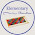## It's Elementary!

### Introducing Fractions

After the key lesson for introducing the concept of fractions we then go on to start providing the language aspect.

For this presentation I use a narrow strip of black paper, labels for 'denominator' and 'numerator' and corresponding number and word cards. I also like to write on strips of paper at the conclusion of the lesson.

Firstly we explain to the child that there are two parts of the fraction to understand. The number under the black line is called the denominator. The denominator is the family name - it tells us how many members belong to that family.

The numerator is above the line and tells us how many of the family are present.

We then produce the corresponding numeral over the denominators:

We then continue with the rest of the three period lesson by asking the children to show the denominator, asking what numerators do, etc. To finish we show the child how we write these:

Revision of this lesson is required before moving on to the next presentation.

You can find a PDF file of the labels that can be used for this lesson, and any extensions, here. After this lesson the children can practice matching them and even writing them if they like.

I hope to make some booklets soon that I can share with you all :)

#### 4 comments:

1.Thank you for this! I'm slooowly figuring out how to teach my students fractions and this is the first lesson I've seen that makes sense!

2.You're welcome :)

3.This is a great lesson! Thank you :)

1.Thanks Cherine :)